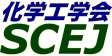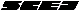## \$B9V1i%W%m%0%i%`!J%;%C%7%g%sJL!K(B

### \$B%7%s%]%8%&%`(B \$B!c:`NA!&3&LLF\$O@2q%]%9%?!<%;%C%7%g%s!V:`NAAO@.\$H3&LL8=>]!W!d(B

#### XA2P41-XA2P84

 \$B%*!<%,%J%\$%6!<(B \$BD9HxBgJe!JElKLBg!K!&>.NSK'CK!J0q>kBg!K!&

\$B:`NA3&LLIt2q\$G\$O!\$3FJ,2J2q\$G\$N]\$K%]%9%?!<>^\$NA*9M\$b9T\$&M=Dj\$G\$"\$k!#B?\$/\$N8&5fe!\$JL8D\$N%7%s%]\$H\$J\$C\$F\$\$\$k\$b\$N\$N!\$It2qF\$O@2q%7%s%]\$H0lBN\$K1?1D\$5\$l\$k\$b\$N\$G\$"\$k!#(B

\$B:G=*99?7F|;~!'(B2012-08-20 15:02:17
\$B9V1i(B
\$B;~9o(B
\$B9V1i(B
\$BHV9f(B
\$B9V1iBjL\!?H/I=\$B%-!<%o!<%I(B\$BJ,N`(B
\$BHV9f(B
\$B\$BHV9f(B
XA\$B2q>l(B \$BBh(B2\$BF|(B
13:00\$B!A(B 15:00XA2P41\$B%j%]%=!<%`Kl\$r!V>l!W\$H\$7\$?(BL-Pro\$B?(G^\$K\$h\$k%"%k%I!<%kH?1~(B
(\$B:eBg1!4p9)(B) (\$B@5(B)\$BGO1[(B \$BBg(B \$B!&(B \$B!{(B(\$B3X(B)\$B@P>e(B \$B6,98(B \$B!&(B (\$B3X(B)\$BF#86(B \$B?5J?(B
Liposome
Aldol reaction
Optical resolution
S-32817
13:00\$B!A(B 15:00XA2P42\$BD>:?>uK0OBC:2=?eAG:?\$rM-\$9\$kN>?FG^@-%^%/%m%b%N%^!<\$N=E9g(B
(\$B:eI\Bg9)(B) \$B!{(B(\$B3X(B)\$BJFED(B \$BNg;K(B \$B!&(B (\$B3X(B)\$B?yK\(B \$B9a?%(B \$B!&(B (\$B@5(B)\$B0BED(B \$B>;90(B \$B!&(B (\$B@5(B)\$B2.Ln(B \$BGn9/(B
macromonomer
micelle
polymerization
S-32178
13:00\$B!A(B 15:00XA2P43\$B%(%^%k%7%g%s%2%k2=K!\$rMQ\$\$\$??75,\$J8GDj2=9ZAGB?9&
(\$BElG@9)Bg1!9)(B) \$B!{(B(\$B3X(B)\$B2O>e(B \$BBnO/(B \$B!&(B (\$B@5(B)\$BFA;3(B \$B1Q><(B
porous gel
immobilized enzyme
lipase
S-32741
13:00\$B!A(B 15:00XA2P44\$B%V%m%C%/%3%]%j%^!<\$NAjJ,N%\$rMxMQ\$7\$?%7%j%+%J%N9=B\$\$N@)8f(B
(\$B;:Am8&(B) \$B!{C]Fb(B \$B9/N4(B \$B!&(B (\$B@5(B)\$BJR2,(B \$B>M(B \$B!&(B \$B;3ED(B \$BK~I'(B \$B!&(B (\$B@5(B)\$B1sF#(B \$BL@(B
block copolymer
phase separation
silica
S-32808
13:00\$B!A(B 15:00XA2P45\$B@\9g:`NAMQ6bB0(BCu\$B%J%NN3;R\$N:n@=\$H@\9gFC@-(B
(\$B0q>kBg9)(B) \$B!{(B(\$B3X(B)\$B0BIt(B \$BM4 \$B!&(B (\$B@5(B)\$B>.NS(B \$BK'CK(B \$B!&(B (\$BF|N)F|N)8&(B) \$BO(B \$B!&(B \$BJ]ED(B \$BM:N<(B
Cu
nanoparticle
metallic bonding
S-32244
13:00\$B!A(B 15:00XA2P46\$B0eNE2hA|?GCGMQ%7%j%+%+%W%;%k2=H/8w@-H>F3BN%J%NN3;R\$N:n@=K!\$N3+H/(B
(\$B0q>kBg9)(B) \$B!{(B(\$B3X(B)\$B>>8M(B \$B42Ip(B \$B!&(B \$BLnBt(B \$BBnLi(B \$B!&(B (\$B@5(B)\$B>.NS(B \$BK'CK(B \$B!&(B (\$BElKLBg1!0e(B) \$BCf@n(B \$BCRI'(B \$B!&(B \$B8"ED(B \$B9,M4(B \$B!&(B \$BBgFb(B \$B7{L@(B
nanoparticles
quantum dots
silica coatiing
S-32319
13:00\$B!A(B 15:00XA2P47\$BN.DL<0H?1~4o\$K\$h\$k9b299b052<\$G\$N6d%J%N%3%m%\$%I\$NO"B3D4@=(B
(\$B;37ABgM}9)(B) \$B!{(B(\$B3X(B)\$B \$B!&(B (\$B3X(B)\$BEDB<(B \$B;J(B \$B!&(B (\$B@5(B)\$B<58M(B \$B>;9-(B
Ag nanoparticle
citric acid
high temperature and pressure
S-32174
13:00\$B!A(B 15:00XA2P48\$B6bB0%J%NN3;RC4;}7?C1J,;6%A%?%K%"8w?(G^N3;R\$N9g@.(B
(\$BElKLBg1!9)(B) \$B!{(B(\$B3X(B)\$B:dK\(B \$B3Y;K(B \$B!&(B (\$B@5(B)\$B@P0f(B \$B<#G7(B \$B!&(B (\$B@5(B)\$BD9Hx(B \$BBgJe(B \$B!&(B (\$B@5(B)\$B:#Ln(B \$B44CK(B
metal nanoparticle
monodisperse titania particle
photocatalyst
S-32480
13:00\$B!A(B 15:00XA2P49\$B%i%a%iAjCr7?\$rMxMQ\$7\$?H,LLBN%"%J%?!<%<(BTiO2\$B7k>=\$N%o%s%9%F%C%W?eG.9g@.(B
(\$BFAEgBg1!@hC<650iIt(B) \$B!{(B(\$B3X(B)\$B<#FA(B \$B=c(B \$B!&(B (\$BFAEgBg9)(B) \$BCf[j(B \$B?p9a(B \$B!&(B (\$BFAEgBg1!@hC<650iIt(B) \$B;38}(B \$BOB4u(B \$B!&(B (\$BFAEgBg1!(BSTS\$B8&(B) (\$B@5(B)\$BCf@n(B \$B7I;0(B \$B!&(B (\$B@5(B)\$B30NX(B \$B7r0lO:(B \$B!&(B (\$B@5(B)\$B?y;3(B \$BLP(B \$B!&(B (\$B@5(B)\$B2CF#(B \$B2mM5(B
titanium oxide
lamellar self-assembly
photocatalysts
S-32605
13:00\$B!A(B 15:00XA2P50\$B%(%l%/%H%m%9%W%l!
(\$B?@8MBg1!9)(B) \$B!{(B(\$B3X(B)\$BM.LZ(B \$BJbH~(B \$B!&(B (\$B3X(B)\$BEZ20(B \$B1G;R(B \$B!&(B (\$B@5(B)\$BF#0f(B \$B><9((B \$B!&(B (\$B@5(B)\$B4];3(B \$BC#@8(B
electrospray
microcapsule
biomineralization
S-321004
13:00\$B!A(B 15:00XA2P51\$B%3%"%7%'%k7?%+!<%\%s!>%A%?%K%"J#9g%U%!%\$%P!<\$N9=B\$@)8f(B
(\$B5~Bg1!9)(B) \$B!{(B(\$B3X(B)\$BEDCf(B \$B\$"\$9\$_(B \$B!&(B (\$B@5(B)\$BD9Nf(B \$B?.Je(B \$B!&(B (\$B@5(B)\$BBgEh(B \$B@5M5(B
Composite Nanofiber
Carbon
TiO2
S-32305
13:00\$B!A(B 15:00XA2P52\$B0eNE2hA|?GCGMQ%3%"(B-\$B%7%'%k7?N3;R\$N3+H/\$K4X\$9\$k8&5f(B
(\$B0q>kBg9)(B) \$B!{(B(\$B3X(B)\$BD9?\(B \$BNK;R(B \$B!&(B \$BCv@%(B \$B908w(B \$B!&(B (\$B@5(B)\$B>.NS(B \$BK'CK(B \$B!&(B (\$BElKLBg1!0e(B) \$BCf@n(B \$BCRI'(B \$B!&(B \$B8"ED(B \$B9,M4(B \$B!&(B \$BBgFb(B \$B7{L@(B
Au
silica-coating
nanoparticle
S-32245
13:00\$B!A(B 15:00XA2P53\$B%^%\$%/%mN.O)\$rMxMQ\$7\$?C1J,;6%3%"(B-\$B%7%'%k7?%j%]%=!<%`\$N@8@.(B
(\$B@iMUBg1!9)(B) \$B!{(B(\$B3X(B)\$B?eLn(B \$B2m7<(B \$B!&(B (\$B@iMUBg9)(B) \$B>.@>(B \$B8+5((B \$B!&(B (\$B@iMUBg1!9)(B) (\$B@5(B)\$B;3ED(B \$B??@!(B \$B!&(B (\$BElBg1!AmJ8(B) \$BK-ED(B \$BB@O:(B \$B!&(B (\$B@iMUBg1!9)(B) (\$B@5(B)\$B4X(B \$B
liposome
non-equilibrium droplet
core-shell
S-32813
13:00\$B!A(B 15:00XA2P54\$B%U%'%i%\$%H\$NHyJ4:U\$K\$h\$k%J%N%5%\$%:<'@-BN\$ND4@=(B
(\$B;37ABg1!M}9)(B) \$B!{(B(\$B3X(B)\$BNkLZ(B \$BM&J?(B \$B!&(B (\$B@5(B)\$BLZKs(B \$B8w@5(B \$B!&(B (\$B@5(B)\$BD9C+@n(B \$B@/M5(B
Magnetic propert
Ferrite
Fine grinding
S-32497
13:00\$B!A(B 15:00XA2P55\$BA*BrE*%Q%k%9%l!<%6!<2CG.\$K\$h\$k0[7?HyN3;R\$N:n@=(B
(\$B;37ABg1!M}9)(B) \$B!{(B(\$B3X(B)\$BJ!ED(B \$B>?(B \$B!&(B (\$B@5(B)\$BHu8}(B \$B7r;V(B \$B!&(B (\$B@5(B)\$BPrED(B \$BCi90(B
Pulse laser
Selective heating
Nonspherical particles
S-32362
13:00\$B!A(B 15:00XA2P56\$BL55!%J%N%3%"\$H%]%j%^!<\$+\$i\$J\$k0[J}@-J#9gN3;R\$N9g@.(B
(\$BElKLBg1!9)(B) \$B!{(B(\$B3X(B)\$B]/0f(B \$BM*(B \$B!&(B (\$B@5(B)\$B@P0f(B \$B<#G7(B \$B!&(B (\$B@5(B)\$BD9Hx(B \$BBgJe(B \$B!&(B (\$B@5(B)\$B:#Ln(B \$B44CK(B
anisotropic particle
nanocomposite
silica core
S-32451
13:00\$B!A(B 15:00XA2P57\$BEE>l0u2C\$rMxMQ\$7\$?0[J}@-N3;R\$NEE6K>e\$X\$NG[8~=8@Q(B
(\$BElKLBg1!9)(B) \$B!{(B(\$B3X(B)\$BCi(B \$B@.EM(B \$B!&(B (\$B@5(B)\$B@P0f(B \$B<#G7(B \$B!&(B (\$B@5(B)\$BD9Hx(B \$BBgJe(B \$B!&(B (\$B@5(B)\$B:#Ln(B \$B44CK(B
anisotropic particle
electric field
orientation
S-32436
13:00\$B!A(B 15:00XA2P58\$BKlI=LL\$NA_\$-
(\$B6e9)Bg9)(B) \$B!{(B(\$B3X(B)\$B:{ED(B \$B63J?(B \$B!&(B (\$B@5(B)\$B;3B<(B \$BJ}?M(B \$B!&(B (\$B@5(B)\$BGOEO(B \$B2B=((B \$B!&(B (\$B@5(B)\$B \$B!&(B (\$B;q@8F2%j%5!<%A%;%s%?!<(B) \$BA>2fIt(B \$BFX(B
heat flux method
drying
coating
S-32827
13:00\$B!A(B 15:00XA2P59ZnO\$B%J%N%m%C%IGvKl>e\$X\$N6bB05Z\$S6bB0;@2=J*H>F3BN\$NEE2r@O=P5sF0(B
(\$B;37ABg1!M}9)(B) \$B!{(B(\$B3X(B)\$B9b66(B \$BBgJe(B \$B!&(B (\$B3X(B)\$B@6?e(B \$B=(N4(B \$B!&(B (\$B@5(B)\$BHu8}(B \$B7r;V(B \$B!&(B (\$B@5(B)\$BPrED(B \$BCi90(B
ZnO
Nanorod
Electrodeposition
S-32261
13:00\$B!A(B 15:00XA2P60\$B%0%i%U%H%]%j%^!<\$H%7%i%s%+%C%W%j%s%0;nLt\$K\$h\$k6bB0EAG.LL\$N?F?e2=(B
(\$B1'ET5\Bg1!9)(B) \$B!{(B(\$B3X(B)\$B:4F#(B \$BM\$(B \$B!&(B (\$B1'ET5\Bg9)(B) \$B8EB<(B \$BD>?M(B \$B!&(B (\$B1'ET5\Bg1!9)(B) (\$B@5(B)\$B:4F#(B \$B@5=((B \$B!&(B (\$B@5(B)\$B8E_7(B \$B5#(B \$B!&(B (\$B@5(B)\$BNkLZ(B \$B>:(B \$B!&(B (\$B \$B!&(B \$B>.Ln(B \$BD> \$B!&(B (\$B;:Am8&(B) (\$B@5(B)\$B0\$It(B \$B@kG7(B \$B!&(B (\$B90A0BgM}9)(B) \$BO<(B \$B9LFs(B \$B!&(B (\$B%*!<%S%?%k%(%s%8%K%"%j%s%0(B) \$B:XF#(B \$B2m5,(B \$B!&(B (JAXA) \$B2F0f:d(B \$B@?(B
surface wetting control
graft polymer
heat transfer surface
S-32447
13:00\$B!A(B 15:00XA2P61\$B\$O\$,\$l\$J\$\$3&LL3h@-:^\$K\$h\$kI=LL2~
(\$B?@8MBg1!9)(B) \$B!{(B(\$B3X(B)\$BK\>1(B \$B?rJ8(B \$B!&(B (\$B3X(B)\$BJuF@(B \$B0l5.(B \$B!&(B \$BFn(B \$B=(?M(B \$B!&(B (\$B@5(B)\$B4];3(B \$BC#@8(B
polymerizable surfactant
surface modification
carbon nanotubes
S-32507
13:00\$B!A(B 15:00XA2P62\$BEII[7?9bJ,;R\$K\$h\$k%W%i%9%A%C%/I=LL\$N5!G=2=(B
(\$B?@8MBg1!(B) \$B!{(B(\$B3X(B)\$B;3K\(B \$B=SJe(B \$B!&(B (\$B3X(B)\$B2 \$B!&(B (\$B@5(B)\$B@>Ln(B \$B9'(B \$B!&(B (\$B@5(B)\$B4];3(B \$BC#@8(B
plastic
biosensor
surface modification
S-32711
13:00\$B!A(B 15:00XA2P63\$B%P%s%/4pHD>e\$K7A@.\$7\$?DcJ,;RM-5!(BEL\$BGvKl7A>u\$HA`:n>r7o\$N4X78(B
(\$B6eBg1!9)(B) \$B!{(B(\$B3X(B)\$B86ED(B \$B9I?-(B \$B!&(B (\$B6eBg(BOPERA) \$B:d>e(B \$B7C(B \$B!&(B (\$B6eBg9)(B) (\$B@5(B)\$B?<0f(B \$B=a(B \$B!&(B (\$B6eBg(BOPERA) \$B0BC#(B \$B@iGHLp(B
OLED
thin film
film shape
S-32929
13:00\$B!A(B 15:00XA2P64\$B%*%l%\$%s;@7O%Y%7%/%k\$N?6F0E*9=B\$JQ2=(B
(\$BF1;VOB(B \$B0&Mx9a(B \$B!&(B (\$BF1;VO5W(B
vesicle
sodium oleate
periodic structural change
S-3269
13:00\$B!A(B 15:00XA2P65\$B;i
(\$BC^GHBg1!@84D2J(B) \$B!{(B(\$B3X(B)\$B@>ED(B \$BM39aN\$(B \$B!&(B (\$B3X(B)\$B>.NS(B \$BM59,(B \$B!&(B (\$BEl5~;TBg9)(B) (\$B@5(B)\$B9u4d(B \$B?r(B \$B!&(B (\$BC^GHBg1!@84D2J(B) (\$B@5(B)\$B:4F#(B \$B@?8c(B \$B!&(B (\$B@5(B)\$B;T@n(B \$BAO:n(B
giant vesicle
flow cytometric analysis
microencapsulation
S-32574
13:00\$B!A(B 15:00XA2P66\$B;iKC;@%Y%7%/%k\$NKlFC@-\$*\$h\$S%(%9%F%k2C?eJ,2rH?1~\$N2r@O(B
(\$B:eBg1!4p9)(B) (\$B@5(B)\$BGO1[(B \$BBg(B \$B!&(B \$B!{(B(\$B3X(B)\$B2#0f(B \$BCR:H(B \$B!&(B (\$B@5(B)\$BEgFb(B \$B
Fatty acid
Vesicle
Hydrolysis
S-32777
13:00\$B!A(B 15:00XA2P67\$B?e>=H/?6;R%^%\$%/%m%P%i%s%9(B(QCM)\$BK!\$K\$h\$k%?%s%Q%/
(\$BKL8+9)Bg(B) \$B!{(B(\$B3X(B)\$BLnED(B \$B@E;^(B \$B!&(B (\$B@5(B)\$B?{Ln(B \$B5|(B \$B!&(B (\$B@5(B)\$BKYFb(B \$B=_0l(B
QCM
Adsorption and desorption of BSA
Apatite
S-32734
13:00\$B!A(B 15:00XA2P68\$BN3;RJ,;6Kl4%AgCf\$N7V8w4Q;!\$K\$h\$k%i%F%C%/%9N3;RG;EYJ,I[7WB,(B
(\$B6e9)Bg9)(B) \$B!{(B(\$B3X(B)\$B?98w(B \$BBg \$B!&(B (\$B@5(B)\$B;3B<(B \$BJ}?M(B \$B!&(B (\$B@5(B)\$BGOEO(B \$B2B=((B \$B!&(B (\$B@5(B)\$B
segregation
coating
particle
S-32812
13:00\$B!A(B 15:00XA2P69PSL\$B%J%NN3;R\$NHy@8J*:YK&\$X\$NIUCe!&
(\$B:eI\Bg9)(B) \$B!{(B(\$B3X(B)\$B5\:j(B \$B=`J?(B \$B!&(B \$B5\K\(B \$B825W(B \$B!&(B (\$B@5(B)\$B>.@>(B \$B9/M5(B \$B!&(B (\$B@5(B)\$BLnB<(B \$B=SG7(B
nanoparticle
uptake
S-32464
13:00\$B!A(B 15:00XA2P70\$B%M%*%K%3%A%N%\$%I7O;&Cn:^FbJq%+%W%;%k2=@=:^\$N@=:^@_7W\$,5Z\$\\$9=yJ|@)8f(B
(\$B \$B!&(B (\$B@5(B)\$B5HED(B \$B>;90(B \$B!&(B (\$B@5(B)\$BIp0f(B \$B9'9T(B \$B!&(B (\$B@5(B)\$BBg3Q(B \$B5A9@(B \$B!&(B (\$B@5(B)\$B0&9C(B \$BNC;R(B \$B!&(B (\$BET>k9b@l(B) (\$B@5(B)\$B@6;3(B \$B;KO/(B \$B!&(B (\$B5\:jBg9)(B) (\$B@5(B)\$B1v@9(B \$B900lO:(B
microcapsule
neonicotinoid
controlrelease
S-32800
13:00\$B!A(B 15:00XA2P71\$B%1%i%A%sAH?%:F@8\$N\$?\$a\$NF}@-%?%s%Q%/2A(B
(\$B \$B!&(B (\$B@5(B)\$B5HED(B \$B>;90(B \$B!&(B (\$B@5(B)\$BIp0f(B \$B9'9T(B \$B!&(B (\$B@5(B)\$BBg3Q(B \$B5A9@(B \$B!&(B (\$B@5(B)\$B0&9C(B \$BNC;R(B \$B!&(B (MC\$B%i%\(B) (\$B@5(B)\$BH( \$B!&(B (\$BET>k9)@l(B) (\$B@5(B)\$B@6;3(B \$B;KO/(B \$B!&(B (\$B5\:jBg9)(B) (\$B@5(B)\$B1v@9(B \$B900lO:(B
Microsphere
Keratin
S-32799
13:00\$B!A(B 15:00XA2P72\$B29EY1~Ez@-%]%j%^!<%J%NN3;R\$K\$h\$kG=F0E*%\$%*%sM"Aw(B
(\$B6eBg1!9)(B) \$B!{(B(\$B3X(B)\$BBg66(B \$BNIJ?(B \$B!&(B (\$B@5(B)\$B;01:(B \$B2B;R(B \$B!&(B (\$B@5(B)\$B@1Ln(B \$BM'(B
polymer nanoparticle
thermoresponsive polymer
pH change
S-32567
13:00\$B!A(B 15:00XA2P73\$B3&LLH?1~K!\$rMQ\$\$\$?29EY1~Ez@-%^%\$%/%m%+%W%;%k\$ND4@=\$H29EY1~Ez@-\$K5Z\$\\$9D4@=>r7o\$N1F6A(B
(\$B6e9)Bg9)(B) \$B!{(B(\$B3X(B)\$BGGLn(B \$BfFBg(B \$B!&(B (\$B@5(B)\$BGOEO(B \$B2B=((B \$B!&(B (\$B@5(B)\$B;3B<(B \$BJ}?M(B \$B!&(B (\$B@5(B)\$B
microcapsule
interface riaction
thermo responsive property
S-32925
13:00\$B!A(B 15:00XA2P74\$B4629@-%2%kHyN3;R\$NAB?e@-J*
(\$B9-Bg1!9)(B) \$B!{(B(\$B3X(B)\$B2O@%(B \$B=S2p(B \$B!&(B (\$B@5(B)\$B8eF#(B \$B7rI'(B \$B!&(B (\$B@5(B)\$BGw86(B \$B=\$<#(B
reactive surfactant
bisphenol A
S-32365
13:00\$B!A(B 15:00XA2P75\$BB?9&@-;Y;}BN\$K%0%i%U%H\$7\$?4629@-%]%j%^!<\$K\$h\$k?eCf\$N9EEY@.J,\$N5[C&CeFC@-(B
(\$B9-Bg1!9)(B) \$B!{(B(\$B3X(B)\$B7*;3(B \$BPRM4(B \$B!&(B (\$B@5(B)\$BGw86(B \$B=\$<#(B
thermosensitive polymer
temperature swing
S-32483
13:00\$B!A(B 15:00XA2P76\$B%7%j%+5Z\$SC:AG\$N5[Ce%Q%i%a!<%?\$rMQ\$\$\$?%7%j%+(B-\$BC:AGJ#9g:`NA\$NB?9&9=B\$I>2A(B
(\$B;37ABg1!M}9)(B) \$B!{(B(\$B3X(B)\$BDZ0f(B \$BN6(B \$B!&(B (\$B@5(B)\$BHu8}(B \$B7r;V(B \$B!&(B (\$B@5(B)\$BPrED(B \$BCi90(B
structural evaluation
composite material
porous material
S-32418
13:00\$B!A(B 15:00XA2P77\$BN3;RJ,;67O\$N%(%l%/%H%m%l%*%m%8!<\$K5Z\$\\$9%Q%?!<%sEE6K\$N1F6A(B
(\$B@iMUBg1!9)(B) \$B!{(B(\$B3X(B)\$B9b66(B \$BOBLi(B \$B!&(B (\$B@5(B)\$BW"@%(B \$BM5Fs(B \$B!&(B (\$B@5(B)\$BBgDZ(B \$BBYJ8(B
Electrorheology
pattern electrode
particle suspension
S-32642
13:00\$B!A(B 15:00XA2P78\$B%]%j%(%A%l%s%*%-%7%I\$rE:2C\$7\$?%"%k%3!<%k:.9g7O%J%NN3;RJ,;67O\$NN.F05sF0(B
(\$B@iMUBg1!9)(B) \$B!{(B(\$B3X(B)\$B6b;R(B \$B0&(B \$B!&(B (\$B@5(B)\$BW"@%(B \$BM5Fs(B \$B!&(B (\$B@5(B)\$BBgDZ(B \$BBYJ8(B
shear-thickening
nanoparticle suspension
polyethylene oxide
S-32747
13:00\$B!A(B 15:00XA2P79\$B@8BN9[J*M3MhB?9&FC@-(B
(\$B \$B!&(B (\$B@5(B)\$BCfN\$(B \$BJY(B \$B!&(B (\$B@5(B)\$B9CHe(B \$B7IH~(B
hydroxyapatite
biominerals
humidity conditioning
S-32901
13:00\$B!A(B 15:00XA2P80\$B%<%*%i%\$%HCr7?C:AG\$N?e>x5\$5[Ce%7%_%e%l!<%7%g%s\$*\$h\$S1~MQ2DG=@-\$N8!F\$(B
(\$B5~Bg9)(B) \$B!{(B(\$B3X(B)\$B0pED(B \$B9?B@O:(B \$B!&(B (\$B@5(B)\$BEDCf(B \$B=( \$B!&(B (\$BElKLBgB?858&(B) (\$B@5(B)\$B@>86(B \$BMNCN(B \$B!&(B (\$B@5(B)\$B5~C+(B \$BN4(B \$B!&(B (\$B5~Bg9)(B) (\$B@5(B)\$B5\86(B \$BL-(B
zeolite templated carbon
molecular simulation
S-3286
13:00\$B!A(B 15:00XA2P81A\$B7?%<%*%i%\$%H\$N9g@.\$H%\$%s%/\$X\$N1~MQ(B
(\$B;37ABg1!M}9)(B) \$B!{(B(\$B3X(B)\$BJ?(B \$B3(M|F`(B \$B!&(B (\$B@5(B)\$BLZKs(B \$B8w@5(B \$B!&(B (\$B@5(B)\$BD9C+@n(B \$B@/M5(B
Zeolite A
Hydrothermal synthesis
Dye ink
S-32175
13:00\$B!A(B 15:00XA2P82\$B%7%j%3%s%J%NN3;R\$N(BpH\$B1~Ez@-6E=8!&J,;6@)8f\$K\$h\$k(BDDS\$B\$X\$N1~MQ(B
(\$BElBg1!9)(B) \$B!{(B(\$B3X(B)\$BLpB<(B \$B7rB@O:(B \$B!&(B (\$B3X(B)\$BB@ED(B \$B@?0l(B \$B!&(B (\$B@5(B)\$B0p_7(B \$B?8(B \$B!&(B (\$B@5(B)\$B;38}(B \$BM34tIW(B
Drug Delivery System
nanoparticle
silicon
S-32639
13:00\$B!A(B 15:00XA2P83\$B%b%k%?%k\$H(BFRP\$BGQ:`\$rMQ\$\$\$?F)?e@-:`NA\$N:n@=\$H@-G=I>2A(B
(\$BElM}Bg1!Am2=(B) \$B!{(B(\$B3X(B)\$BBg@>(B \$BG+?M(B \$B!&(B (\$BElM}Bg9)(B) (\$B@5(B)\$B>.NS(B \$BBgM4(B \$B!&(B (\$B@5(B)\$B9b66(B \$BCR51(B \$B!&(B (\$B@5(B)\$BBgC](B \$B>!?M(B \$B!&(B (\$B@5(B)\$B>1Ln(B \$B8|(B
Fiber Reinforced Plastics
Concrete
Recycle
S-32529
13:00\$B!A(B 15:00XA2P84\$B%9%W%l!<<0:.9g%7%9%F%`\$rMQ\$\$\$?%o%C%/%97|By1U\$ND4@=(B
(\$BElM}Bg1!Am2=(B) \$B!{(B(\$B3X(B)\$BEDCf(B \$B:LfF(B \$B!&(B (\$BElM}Bg9)(B) (\$B@5(B)\$B>.NS(B \$BBgM4(B \$B!&(B (\$B@5(B)\$B9b66(B \$BCR51(B \$B!&(B (\$B@5(B)\$BBgC](B \$B>!?M(B \$B!&(B (\$B@5(B)\$B>1Ln(B \$B8|(B
Spray
Suspension
Wax
S-32527

\$B9V1i%W%m%0%i%`(B
\$B2=3X9)3X2q(B \$BBh(B44\$B2s=)5(Bg2q(B(C) 2012 \$B8x1W
Most recent update: 2012-08-20 15:02:17
For more information contact \$B2=3X9)3X2qElKL;YIt(B \$BBh(B44\$B2s=)5(Bg2q(B \$BLd\$\$9g\$;78(B
E-mail: inquiry-44fwww3.scej.org
This page was generated byeasp 2.28; proghtml 2.28c (C)1999-2011 kawase